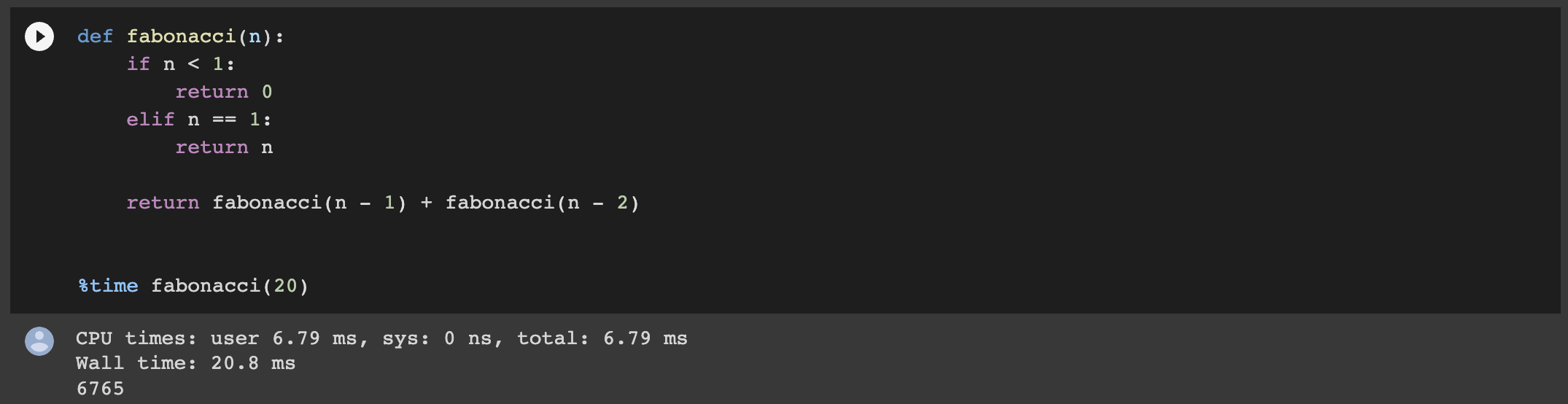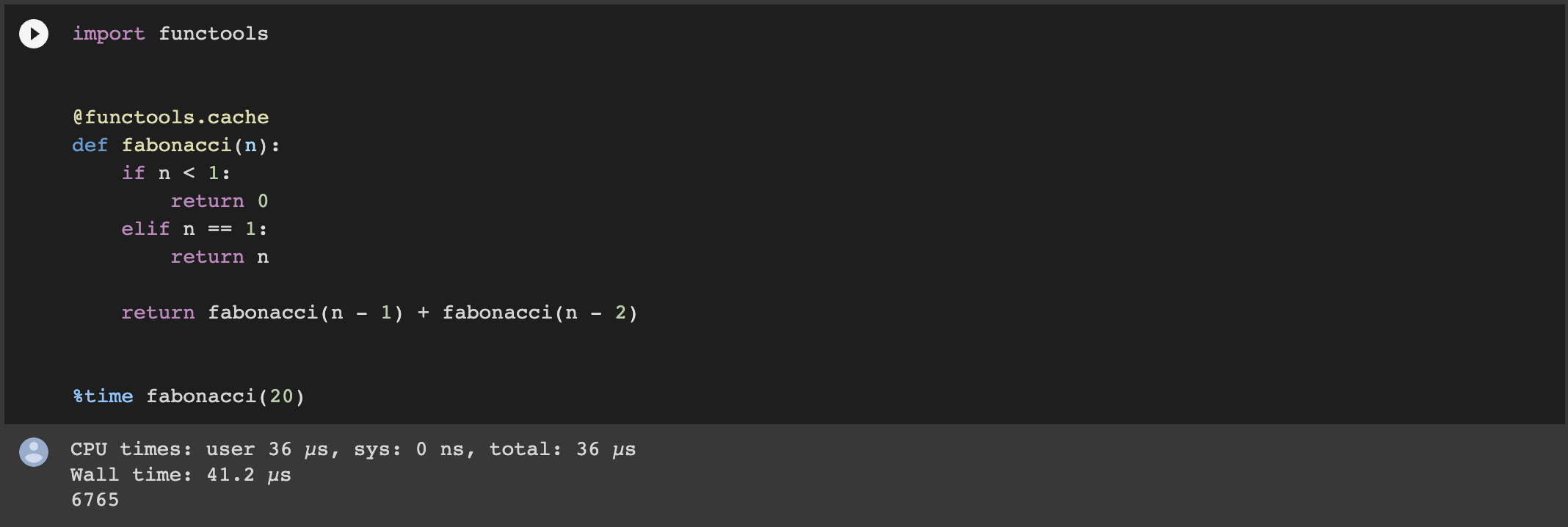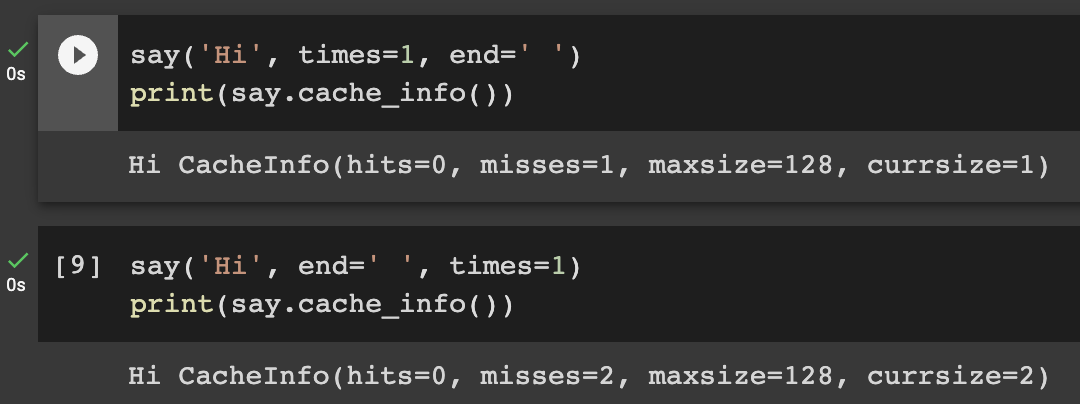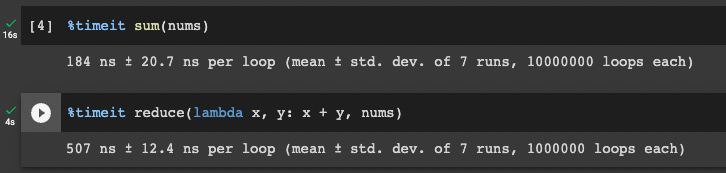# 你應該要知道的 Python 實用模組 - functools 教學

Posted on  Apr 10, 2023  in  Python 程式設計 - 中階  by  Amo Chen  ‐ 6 min read

functools 模組是 Python 內建專門提供各種實用裝飾子(decorator)以及實用函式(function)的模組。

functools 模組最常被使用的功能主要為：

### 本文環境 #

• Python 3.7 以上

### @cache #

``````from functools import cache

@cache
def the_function_you_want_to_cache():
pass
``````

``````def fabonacci(n):
if n < 1:
return 0
elif n == 1:
return n

return fabonacci(n - 1) + fabonacci(n - 2)
````````````from functools import cache

@cache
def fabonacci(n):
if n < 1:
return 0
elif n == 1:
return n

return fabonacci(n - 1) + fabonacci(n - 2)

fabonacci(20)
``````The cache is threadsafe so the wrapped function can be used in multiple threads.

p.s. `@cache` 是 Python 3.9 之後新增的裝飾子

### @lru_cache #

`@cache` 的底層其實使用的就是 `@lru_cache` , `lru`Least Recently Used 的縮寫，`@cache` 等同於 `@lru_cache(maxsize=None)` , 也就是沒有快取筆數限制的 `@lru_cache`

`@lru_cache` 預設大小為 128, 可以快取 128 筆的快取，同樣以 Fabonacci 函式為例，下列範例以參數 `maxsize` 設定大小 32 筆的 cache:

``````from functools import lru_cache

@lru_cache(maxsize=32)
def fabonacci(n):
if n < 1:
return 0
elif n == 1:
return n

return fabonacci(n - 1) + fabonacci(n - 2)

fabonacci(20)
``````

``````>>> fabonacci.cache_info()
CacheInfo(hits=18, misses=21, maxsize=32, currsize=21)
``````

#### 使用 functools 快取相關裝飾子時 2 點注意事項 #

##### 呼叫被快取的函式參數順序 #

``````from functools import lru_cache

@lru_cache()
def say(word, times=1, end='\n'):
for _ in range(times):
print(word, end=end)
``````##### 呼叫被快取的函式參數值必須是 hashable #

``````from functools import lru_cache
from dataclasses import dataclass

@dataclass
class User:
id: int
name: str

@lru_cache()
def query_user_txs(user):
pass

txs = query_user_txs(User(1, 'Jimny'))
``````

``````TypeError Traceback (most recent call last)
<ipython-input-17-dbd843064974> in <cell line: 16>()
14
15
---> 16 txs = query_user_txs(User(1, 'Jimny'))

TypeError: unhashable type: 'User'
``````

``````from functools import lru_cache
from dataclasses import dataclass

@dataclass(frozen=True)
class User:
id: int
name: str

@lru_cache()
def query_user_txs(user):
pass

txs = query_user_txs(User(1, 'Jimny'))
``````

### partial #

Return a new partial object which when called will behave like func called with the positional arguments args and keyword arguments keywords.

partial 函式可以將 1 個函式(function)事先填好部分的參數(arguments args 以及 keyword arguments)，並包裝成 1 個 partial object 回傳，這個 partial object 就像函式一樣可以被呼叫，只是某些參數已經被事先填好，我們只需要帶入剩下所需的參數即可，下列範例以 requests.get 為例，用 partial 函式是先填好 HTTP 標頭(headers), 使用 partial object 時只要填 URL 即可：

``````from pprint import pprint
import requests
import functools

get = functools.partial(
requests.get,
)

pprint(resp.json())
``````

partial 函式很適合對某些函式庫進行再包裝的情況，舉 requests 模組為例，我們可以將某些常用標頭（例如 User-Agent, Accept, Authorization 等）都先用 partial 函式包裝過一次，變成適合呼叫內部系統使用的函式庫，例如：

``````import requests
from functools import partial

'User-Agent': 'Internal System',
'Accept': 'application/json',
'Authorization': 'Basic thesecretekey',
}

internal_get = partial(
requests.get,
)

internal_post = partial(
requests.post,
)

internal_put = partial(
requests.put,
)

internal_patch = partial(
requests.patch,
)

internal_delete = partial(
requests.delete,
)
``````

#### partial, partial 再 partial #

``````def partial(func, /, *args, **keywords):
def newfunc(*fargs, **fkeywords):
newkeywords = {**keywords, **fkeywords}
return func(*args, *fargs, **newkeywords)
newfunc.func = func
newfunc.args = args
newfunc.keywords = keywords
return newfunc
``````

If more arguments are supplied to the call, they are appended to args. If additional keyword arguments are supplied, they extend and override keywords.

``````get = partial(requests.get, headers={'User-Agent': 'Chrome'})
print(get)
get = partial(get, params={'param1': 'p1'})
print(get)
``````

``````functools.partial(<function get at 0x7f40662a9820>, headers={'User-Agent': 'Chrome'})
functools.partial(<function get at 0x7f40662a9820>, headers={'User-Agent': 'Chrome'}, params={'param1': 'p1'})
``````

``````get = partial(get, params={'param2': 'p2'})
print(get)
``````

``````functools.partial(<function get at 0x7f40662a9820>, headers={'User-Agent': 'Chrome'}, params={'param2': 'p2'})
``````

### reduce #

reduce 函式不是裝飾子，而是用來將 iterable 運算縮減成 1 個值的函式，舉下列加總 0 到 4 為例，就是一個典型的 reduce 例子，數字 0 到 4 最後被加總成 1 個值 `total` :

``````nums = [i for i in range(5)]

total = 0
for x in nums:
total += x
``````

``````from functools import reduce

nums = [i for i in range(5)]
reduce(lambda x, y: x + y, nums)
``````

reduce 的第 1 個函式需要接受 2 個值並回傳 1 個值，分別會是 iterable 的前 2 個值，回傳的值就是這 2 個值運算的結果，該運算結果會再被拿來與 iterable 的下一個值丟到同一個函式進行運算，同樣舉數字 0 - 4 總和為例：

``````第 1 次： (0 + 1) = 0

``````

reduce 做的事如果化為數學式 ：

``````((((0 + 1) + 2) + 3) + 4)
``````### @singledispatch #

``````def func(args):
if isinstance(args, int):
print('Got an int', args)
elif isinstance(args, list):
print('Got a list' args)
else:
print('Got something', args)
``````

singledispatch 是 Python 3.4 之後推出的裝飾子，到 3.7 之後變得更加實用，因為該裝飾子到了 3.7 之後支援 Python typing 模組，可以依照參數型別自動呼叫相對應的函式處理。

``````from functools import singledispatch

@singledispatch
def func(args):
print('Got something', args)

@func.register
def _int(args: int):
print('Got an int', args)

@func.register
def _list(args: list):
print('Got a list', args)

func(123)

func([1, 2, 3])

func("123")
``````

``````Got an int 123
Got a list [1, 2, 3]
Got something 123
``````

singledispatch 不僅讓 Python 更容易達到 Single-responsibility principle - Wikipedia, 也增加程式碼的可維護性與簡潔性，是相當方便的裝飾子。

``````@func.register
def _int_or_float(args: int | float):
print('Got an int/float', args)
``````

### 結論 #

Happy Coding!

https://docs.python.org/3/library/functools.html